Create a new printable

Black and white graphics
Color graphics

Select Currency
 US Dollar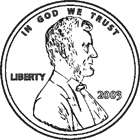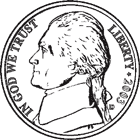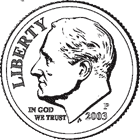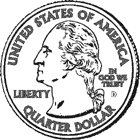Canadian Dollar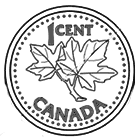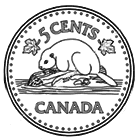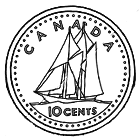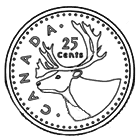Euro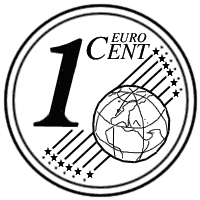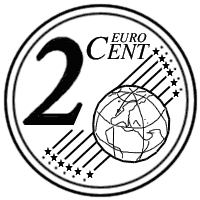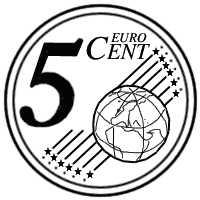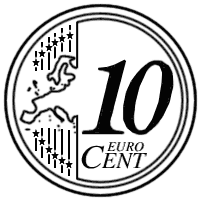Math Worksheets

Sample - Click above to make a new math worksheet (PDF).
 Name _____________________________Date ___________________
Coins
Fill in the missing value.

 1 * This is a pre-made sheet.Use the link at the top of the page for a printable page.
2.
 _____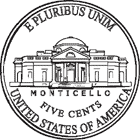+ 1+ 0= 50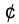_____ nickels  + 1 quarter  + 0 dimes  = 503.
 5+ 1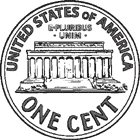+ 3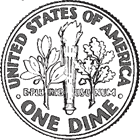= _____5 nickels  + 1 penny  + 3 dimes  = _____4.
 9+ _____+ 4= 949 dimes  + _____ nickels  + 4 pennies  = 945.
 1+ 0+ _____= 411 penny  + 0 dimes  + _____ nickels  = 41Sample
This is only a sample worksheet.

Create a new printable
 US DollarCanadian DollarEuro